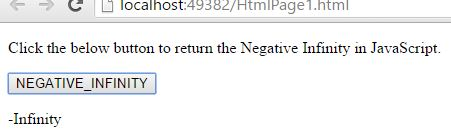# NEGATIVE_INFINITY Property in JavaScript

##### How to return negative infinity in JavaScript?

By using `NEGATIVE_INFINITY `property we can return the negative infinity in JavaScript.

```<p>Click the below button to return the Negative Infinity in JavaScript.</p>
<input type="button" value="NEGATIVE_INFINITY" onclick="Function()" />
<p id="myId"></p>

<script>
function Function() {
document.getElementById("myId").innerHTML = Number.NEGATIVE_INFINITY;
}
</script>
```

In the above code snippet we have given `Id` as "`myId`"to the second `<p>` element in the HTML code, we need to return the negative infinity in JavaScript. For that we are using the code `Number.NEGATIVE_INFINITY`. There is a Function() in the`<script>`block which is connected to the Onclick of the HTML button. The `document.getElementById(`"myId"`) ` returns the element that has Id "id=myID" in the HTML page. Onclick of the button "NEGATIVE_INFINITY" fires the Function() in the `<script>` block at the same time `Number.NEGATIVE_INFINITY `returns the negative infinity in JavaScript and gives the output as "-Infinity".

OUTPUTNOTE: NEGATIVE_INFINTY is considered as lower than any other number.

Views: 3272 | Post Order: 84